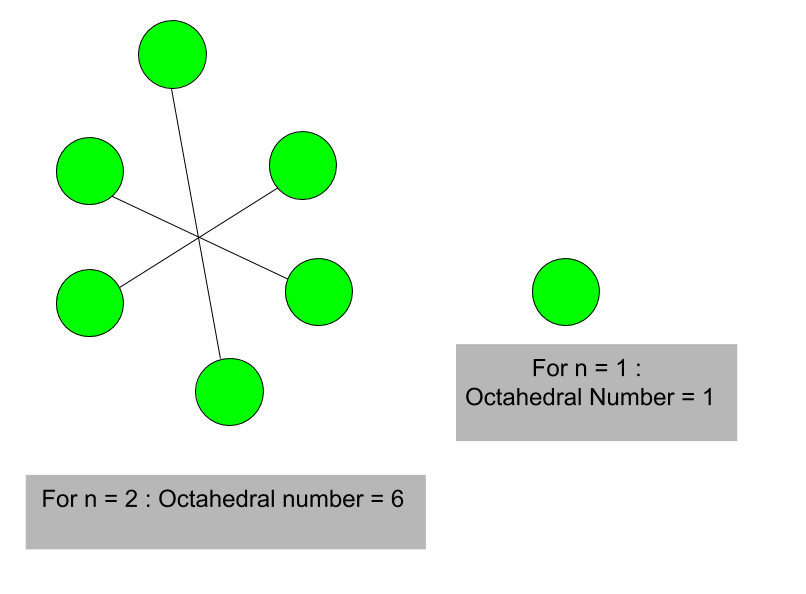Related Articles

# Octahedral Number

• Last Updated : 12 Apr, 2021

Given a number n, the task is to find n-th octahedral number.
A octahedral number belongs to a figurate number and it is the number of spheres in an octahedron built from closely packed spheres. First few octahedral numbers (where n = 0, 1, 2, 3…….) are : 0, 1, 6, 19 and so on.
Examples :

Input : 4
Output : 44
Input : 8
Output : 344Formula for nth octahedral number:

`n * (2n2+1) / 3`

## C++

 `// C++ program to find nth``// octahedral number``#include ``using` `namespace` `std;` `// Function to find``// octahedral number``int` `octahedral_num(``int` `n)``{``    ``// Formula to calculate nth``    ``// octahedral number``    ``return` `n * (2 * n * n + 1) / 3;``}` `// Drivers code``int` `main()``{``    ``int` `n = 5;` `    ``// print result``    ``cout << n << ``"th Octahedral number: "``;``    ``cout << octahedral_num(n);``    ``return` `0;``}`

## Java

 `// Java program to find nth octahedral``// number``import` `java.io.*;` `class` `GFG {` `    ``// Function to find octahedral number``    ``static` `int` `octahedral_num(``int` `n)``    ``{` `        ``// Formula to calculate nth``        ``// octahedral number``        ``// and return it into main function.``        ``return` `n * (``2` `* n * n + ``1``) / ``3``;``    ``}` `    ``// Driver Code``    ``public` `static` `void` `main(String[] args)``    ``{``        ``int` `n = ``5``;``        ``// print result``        ``System.out.print(n + ``"th Octahedral"``                         ``+ ``" number: "``);``        ``System.out.println(octahedral_num(n));``    ``}``}`

## Python3

 `# Python 3 program to find nth``# octahedral number` `# Function to find``# octahedral number``def` `octahedral_num(n) :``    ` `    ``# Formula to calculate nth``    ``# octahedral number``    ``return` `n ``*` `(``2` `*` `n ``*` `n ``+` `1``) ``/``/` `3` `# Driver Code``if` `__name__ ``=``=` `'__main__'` `:``        ` `    ``n ``=` `5``    ``print``(n,``"th Octahedral number: "``                ``, octahedral_num(n))` `# This code is contributed ajit.`

## C#

 `// C# program to find nth``// Octahedral number``using` `System;` `class` `GFG``{``    ` `    ``// Function to find``    ``// octahedral number``    ``static` `int` `octahedral_num(``int` `n)``    ``{` `        ``// Formula to calculate``        ``// nth octahedral number``        ``// and return it into``        ``// main function.``        ``return` `n * (2 * n *``                    ``n + 1) / 3;``    ``}` `    ``// Driver Code``    ``static` `public` `void` `Main ()``    ``{``        ``int` `n = 5;``        ` `        ``// print result``        ``Console.Write(n + ``"th Octahedral"``                        ``+ ``" number: "``);``        ``Console.WriteLine(octahedral_num(n));``    ``}``}` `// This code is Contributed by m_kit`

## PHP

 ``

## Javascript

 ``

Output :

`5th Octahedral number: 85`

Attention reader! Don’t stop learning now. Get hold of all the important DSA concepts with the DSA Self Paced Course at a student-friendly price and become industry ready.  To complete your preparation from learning a language to DS Algo and many more,  please refer Complete Interview Preparation Course.

In case you wish to attend live classes with experts, please refer DSA Live Classes for Working Professionals and Competitive Programming Live for Students.

My Personal Notes arrow_drop_up